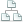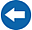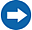Home >Tips >Excel 2016 from Scratch >Calculate sums BSite map

# Excel 2016 from Scratch Exercise Calculate Sums B

## An Excel tutorial by Peter KalmstromIn this demo in the Excel 2016 from Scratch series Peter Kalmstrom explains how to calculate sums by either using the AutoSum feature or selecting the cells which have values that should be calculated.

Peter also shows how to drag formulas with relative references to other cells. He uses the sales figures for different kinds of brushes sold under each quarter as an example in this exercise.

### Exercise

If you want to try the exercise yourself, please download the Excel file Peter uses in the demo!

### Content

This is what Peter shows in the demo below:

• How to create AutoSum formulas and select the cells to include.
• How to create a formula that calculates a sum by selecting cells for the calculation.
• How to give a general format to cells.
• How to format number cells to have a thousand separator.
Peter uses Excel 2016 for his demo, but the Excel basics are the same for earlier versions of Excel.